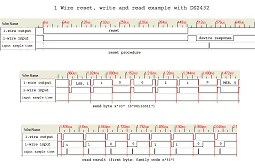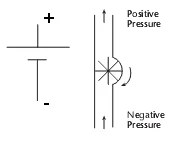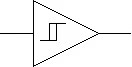## 1-Wire protocol simple and easy1-Wire communication protocol was developed by Dallas Semiconductor owned by Maxim. This protocol allows communication of multiple chips to one host with minimal pin count. The protocol is called 1-Wire because it uses 1 wire to transfer data. 1-Wire architecture uses pull up resistor to pull voltage of data line at master side. 1-Wire protocol uses CMOS/TTL logic and operates at supply voltage range 2.8 to 6V. Master and slave can be receivers and transmitters, but only one direction at a time. LSB goes first always. Data in 1-wire network is is transferred by time slots. For instance to write logic â€œ1â€, master pulls the bus low for 15us or less. To write logic â€œ0â€ master pulls buss low for at least 60us. System clock is not required as each part is self clocked and synchronized by faling edge of master.   Before communicating master resets network by holding the bus low for at least 480us and then looking for responding pulse from slaves. Then master calls slave by its address which is unique for each slave device. Each device has 48 bit (six bytes) globally unique address where last eight bits are CRC of first 56 bits. First byte…

## Avoid noises in mixed signal designToday most of all embedded systems consist of two part circuitry â€“ digital and analog. Digital part is usually controller, its timing circuit and other input output devices. Frequently there is an analog part on same board like ADC, OP amplifiers, sensors and other analog circuitry. Such designs are called mixed-signal designs. Where digital and analog part meats â€“ the grounding problems starts. Fact is that each conductor has its own impedance, so any current flowing result in voltage drops. Ground wires and planes isn’t exceptions. Digital and analog grounds can generate significant electromagnetic radiation that adds noises to signals we need. So the over all system quality drops because o poor design.   In a good design analog ground palane and digital ground plane should be separated. With multilayer PCB this can be done very easy. Another issue is that digital signal traces shouldn’t cross analog ground and analog signal wires shouldn’t cross digital ground plane area. Well of course and try to avoid aligning digital and analog wires as they can catch each other radiated noise. How to deal with these problems. Well first thing is to recognize problematic areas correctly. Then you will be able to implement…

## Calculating of electrical heating elements

Electrical heating elements are often used for teapots, irons, electric ovens, soldering irons etc. When projecting designs with electric heating elements you need to do some calculations that may seem difficult at first glance. But when looking more deeply this becomes simple task. We know that electric heating is a result of current flow in wire with some resistance. Resulting heat is work done by electric current. Work A(J) can be calculated by formula: A=UÂ·IÂ·t Where U â€“ Voltage(V), I â€“ current(A), t â€“ time(s). Then amount of heat produced in wire where electric current flows is calculated as follows: Q=I2Â·RÂ·t Lets calculate simple problem. How much time we need to boil 2 liters of water? Lets take voltage U=220V; Heating element requires current I=4A; Efficiency coefficient is 80%; Starting temperature of water is 20Â°C; Water specific heat â€“ 4200(kJ/kgK). First we have to calculate the amount of heat required to boil a water: Qn=CÂ·mÂ·(tboil-t0)=4200Â·2Â·(100-20)=672000J; With efficiency of 80% we get that wee neet to produce Q=Qn/Î·=672000/0.8=840000J. So we get that Q=A=UÂ·IÂ·t where time needed to boil: t=Q/(UÂ·I)=840000/(220Â·4)=954s=15min and 54s. When we know work produced by current during time period â€“ we can find the power: P=A/t = UI =…

## Calculating wire diameter for maximum current

When selecting wire diameter we usually lok for cross reference tables where you can find recommended wire diameter for maximum current drive. But sometimes may be more useful to calculate by formula than look in to the tables. This way you can have more accurate results. There is nothing new just simple physics. Wire resistance (Î©)is calculated as follows: R = ÏÂ·l/S or R = (1.27Â·ÏÂ·l )/d2; Where Ï â€“ resistivity of material (Î©Â·m), found in tables, l â€“ wire length (m), S â€“ cross selection area(m2), d – wire diameter (m). According to this we can calculate wire length, when other parameters are known: l = RÂ·S/Ï or l = (0.785Â·RÂ·d2)/Ï Cross selection area can by found as follows: S=0.785Â·d2. Wire resistance depends on temperature. Lets say R2 is resistance for temperature t2 and R1 is for t1(usually t1 = 18Â°C), then: R2=R1Â·(1+Î±Â·(t2-t1)) Where Î± â€“ temperature coefficient (K-1). Lets say that maximum current for cross selection area is marked as Î” (A/mm2), then maximal current can be found I=0.785Â·Î”Â·d2. Needed diameter can be found by formula(âˆš – square root symbol): d=âˆš((1.27Â·I)/Î”) So if allowed load is Î”=2A/mm2, then d=0.8âˆšI. If diameter is les than 0.2mm, then melting current…

## Electric circuits analogy to water pipesSometimes calculating parameters and behaviour of some objects may be much easier when using analogy to objects with well developed theory and calculation methodology. In earlier article we analysed power dissipation of electronic devices using Ohms law where Voltage=temperature, Current=Dissipation and Resistance=Thermal resistance. This time lets look how can electronic devices transformed in water pipes and vice versa. Lets take Voltage source. A simple battery is like a water pump which provides a Pressure (a voltege analogy): Then second electronic element is a resistor. Resistor can be imagined as water pipe with smaller aperture. The higher resistance is â€“ the smaller aperture of pipe: Now we have the elements needed for constructing a simple circuit. We have Pressure = Voltage, Resistance=Flow resistance of pipe. Current = Water flow. The electric current and water flow can be calculated using the same Ohms Law formula: I=V/R In reality there are many limitations of such approach as operating temperatures, power dissipation and power limits. But with some corrections to model calculations can be quite accurate. Ok lets see how semiconductors can be converted to water pipe system elements. Diode is imagined as valve allowing current flow to one direction. In this case diode…

## Thermal analysis of semiconductor devices-when do you need heat-sinks

Every electronics constructor and engineer knows that electronic devices like IC’s voltage regulators and CPU heats up. Temperature is critical parameter for reliable system operation. System reliability is very closely related to device temperature high or low. While temperature increases the reliability drops exponentially. If you look in device datasheets you will find a recommended Operating temperature range. For instance Voltage regulator 7805 can normally operate at 0 – 70°C temperature range. When maximum Junction Temperature can reach up to 150°C. So how is operating temperature range calculated. It is not as difficult as it may look like. We know, that temperature is analogous to voltage, thermal resistance changed to electric resistance, power dissipation is as current, then: Temperature=Power*Thermal_Resistance; Temperature means the rise in °C, Power in Watts and Thermal_Resistance (°C/Watts). The calculation can be done using simple Ohms law. Lets analyse real example by using simple 7805 voltage regulator. We know that output produces 5V. Lets assume that input is 9V and output provides 1A current. Then regulators power dissipation is: P=V·I=(9V-5V)·1A=4 Watts So datasheet says that thermal resistance of junction to case (TO-220) is 4°C/W and case(without heat-sink) to ambient is 50°C/W. Then total thermal resistance is Rt=4+50=54°C/W.…

## Schmitt trigger VS simple inverterSchmitt trigger is special logical element adjusted to work with analog input signals. The primary purpose of Schmitt triggers were restoring the shape of digital signals. Because of transmission line effect digital shape transforms from square to trapezoid or triangle or more complex signal. Of course during transmission signals become noisy and distorted. Schmitt trigger is a comparator circuit but inside it is a positive feedback what results in hysteresis and memory effect. Comparing to simple logical elements Schmitt triggers have two threshold levels. Between these threshold values U1 and U2 the state of output doesn’t change what is so called a hysteresis. Such effect stabilizes output against rapid triggering by noise. Bellow is two characteristics compared of simpel logical inverter and Schmitt trigger inverter: We see that Schmitt trigger characteristics is more complex -the output signal threshold depends on direction of input voltage rise/drop. When input signal is rising towards Ucc, then Threshold is U2, when input voltage is dropping towards zero- threshold become U1. Lets see how this would look with real noisy signal. Because of hysteresis any noise that amplitude is lower that U2-U1 is removed. And another fact, that signal front becomes square. Standard digital chips…